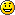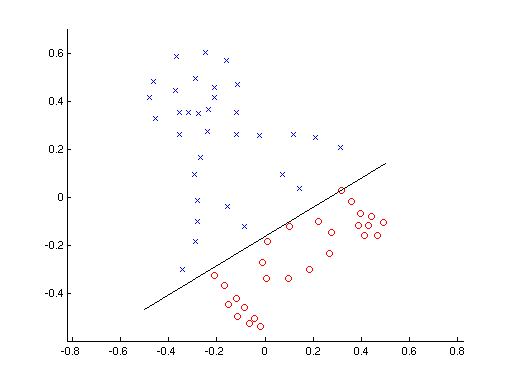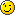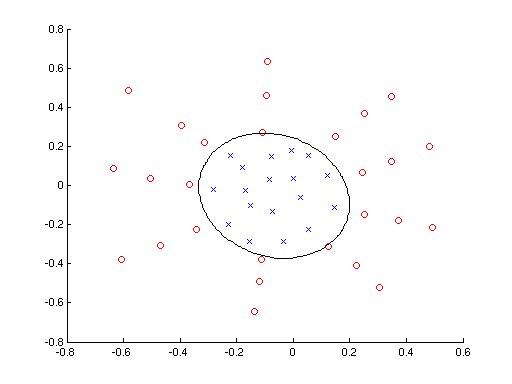Warning

# Perceptron Algorithm

In all the previous labs, we were trying to design a classifier $q(\mathbf{x}) : \mathcal{X} \rightarrow D$ by using the a priori probabilities $p_K(k)$ and the probability density functions $p_{X|k}(x|k)$. We were minimising the Bayesian risk or some more complex criterion. This kind of approach is commonly referred to as generative, because we try to estimate the full probabilistic model of all variables (we try to find the probabilistic model which “generated” the training samples).

Sometimes, this is however not practical or even possible, e.g. it is not possible to get enough samples to get a good estimate of $p_{X|k}(\mathbf{x}|k)$, so instead we try to approximate the decision function $q(\mathbf{x})$ directly without any knowledge of the underlying probability distribution. This approach is referred to as discriminative, because we are only interested in training the classifier to make correct decisions and we do not care about the actual probabilistic model.

In the discriminative approach, we define a criterion (emperical risk) $R_{\mbox{emp}}(q)$ which estimates the risk of the classifier using the training set. Typically, we calculate $R_{\mbox{emp}}(q)$ as the classification error of the classifier $q$ on the training set $\mathcal{T}$) and we try to find a classifier $q^*$ which minimizes the emperical risk $$\mathcal{T} = \{(\mathbf{x}_i, y_i); \mathbf{x}_i\in R^n, y_i\in\{1,2\}\}_{i=1,\ldots,N}$$ $$R_{\mbox{emp}}(q) = \frac{1}{N}\sum_{i=1}^N W(q(\mathbf{x}_i), y_i)$$ $$q^* = \arg\min_q R_{\mbox{emp}}(q)$$ where $W$ denotes the loss function (typically the zero-one loss function is used). Note that in the discriminative model task formulation no probability densities are used. In this lab we will also strictly use bold letters for vectors and regular font for scalars.

## Information for development in Matlab

To fulfil this assignment, you need to submit these files (all packed in a single .zip file) into the upload system:

• answers.txt - answers to the Assignment Questions
• assignment_06.m - a script for data initialisation, calling of the implemented functions and plotting of their results (for your convenience, will not be checked)
• perceptron.m - a function which implements the perceptron algorithm
• lift_dimension.m - a mapping function which lifts the dimensionality of the feature space
• classif_quadrat_perc.m - a quadratic discriminative function
• perceptron_linear.png, perceptron_quadratic.png - images specified in the tasks

## Information for development in Python

Experimental. Use at your own risk.

Standard is to use Matlab. You may skip this info box if you follow the standard rules.

To fulfil this assignment, you need to submit these files (all packed in a single .zip file) into the upload system:

• answers.txt - answers to the Assignment Questions
• percept.ipynb - a script for data initialisation, calling of the implemented functions and plotting of their results (for your convenience, will not be checked)
• percept.py - containing the following implemented methods
• perceptron - a function which implements the perceptron algorithm
• lift_dimension - a mapping function which lifts the dimensionality of the feature space
• classif_quadrat_perc - a quadratic discriminative function
• perceptron_linear.png, perceptron_quadratic.png - images specified in the tasks

Use template of the assignment. When preparing the archive file for the upload system, do not include any directories, the files have to be in the archive file root.

## Task 1: The Perceptron Algorithm

Today's task is to implement the perceptron algorithm for learning a linear classifier. In the case of a linear classifier for two classes ($|K|=2$), the discriminative function $q(\mathbf{x}): \mathcal{X} \rightarrow \{1, 2\}$ can be expressed as

$$q(\mathbf{x}) = \left\{ \begin{array}{l l} 1 & \quad \mathbf{x} \cdot \mathbf{w} + b > 0 \\ 2 & \quad \mathbf{x} \cdot \mathbf{w} + b < 0 \end{array} \right.$$

where $\mathbf{x} = (x_1, \ldots, x_n)^T$, $\mathbf{w} = (w_1, \ldots, w_n)^T$, and $\mathbf{x} \cdot \mathbf{w} = \sum_{i=1}^n x_i w_i$ denotes the dot product and the dimensionality of $\mathcal{X}$ is $n$. In geometrical interpretation, the expression $\mathbf{x} \cdot \mathbf{w} + b = 0$ is an equation of a line (or a hyperplane in higher dimensions) which divides the space into two subspaces and the value of the discriminative function $q(\mathbf{x})$ represents in which of the two subspaces the vector $\mathbf{x}$ is located.

Thus, the task of finding a linear classifier for two classes can be formulated as finding parameters $\mathbf{w}$ and $b$ so that the following system of linear inequalities holds for each item $(\mathbf{x}_i, y_i)$ in the training set: $$\mathbf{w} \cdot \mathbf{x}_i + b > 0 \quad \mbox{if}\;y_i = 1$$ $$\mathbf{w} \cdot \mathbf{x}_i + b < 0 \quad \mbox{if}\;y_i = 2$$

The solution of the system exists only if the training data are linearly separable, in which case the solution can be found using the perceptron algorithm.

The system of inequalities can be equally rewritten as $$\mathbf{v} \cdot \mathbf{z}_i > 0$$ if we substitute $$\mathbf{v} = [\mathbf{w}; b]$$ $$\mathbf{z}_i = [\mathbf{x}_i; 1] \quad \mbox{if}\;y_i = 1$$ $$\mathbf{z}_i = -[\mathbf{x}_i; 1] \quad \mbox{if}\;y_i = 2$$ (try it yourself on a piece of paper - all that happened here is that we made a 'clever' substitution so that we still have a system of $N$ inequalities, but now all inequalities have the same format).

Now we can easily describe the algorithm:

The Perceptron Algorithm

1. Initialize $\mathbf{v} = \mathbf{0}$
2. Find a vector $\mathbf{z}_t$ in the (transformed) training set $\{\mathbf{z}_1,\mathbf{z}_2,\ldots,\mathbf{z}_N\}$ for which the inequality $\mathbf{v} \cdot \mathbf{z}_i > 0$ does not hold.
3. If such vector does not exist (i.e. all inequalities are satisfied), terminate the algorithm; the parameters $\mathbf{w}$ and $b$ can be simply extracted from $\mathbf{v}$ because $\mathbf{v} = [\mathbf{w}; b]$. Otherwise, continue.
4. Use the vector $\mathbf{z}_t$ to adapt the solution $$\mathbf{v} := \mathbf{v} + \mathbf{z}_t$$ and go to step 2

1. Create a function [w b] = perceptron(X, y, maxIterations) which implements the perceptron algorithm. The function accepts a matrix X of $N$ training samples and a vector y of training labels. The parameter maxIterations specifies the maximal number of iterations of the algorithm. If a solution is not found in the given number of iterations the function is expected to return [NaN, NaN] to signal this situation.
X = [1 1 2 2; 1 3 5 6];
y = [1 1 2 2];
[w,b] = perceptron(X, y, 100)

w = [2; -3]
b = 9

X = [3 1 2 2; 2 3 5 6];
y = [1 1 2 1];
[w,b] = perceptron(X,y, 100)

w = NaN
b = NaN
2. Test its functionality on synthetic two-dimensional linearly separable data that you create.
• Use the createdata script to manually generate the data
• Find the linear classifier using the perceptron algorithm (the perceptron function you created)
• Visualize the classifier using the pline and ppatterns functions and save the figure as perceptron_linear.png You are encouraged to create an interesting or funny picture of your own3. Test the algorithm for data, which cannot be linearly separated (e.g. the XOR problem). What's happening in this non-separable case?

## Task 2: Lifting dimensionality of the feature space

As we have seen in the last point of the first task, for some data a linear discriminative function that would separate the data does not exist.

In order to deal with this problem, we will extend the perceptron algorithm so that it learns quadratic discriminant function (see ). We define a mapping function $\phi: \mathcal{X} \rightarrow \mathcal{Z}$ which projects the training samples $\mathbf{x} \in \mathcal{X}=\mathbb{R}^2$ into a higher-dimensional space $\mathcal{Z}$.

In our task, we will consider the discriminative function in the form $$q'(\mathbf{x}) = w_1x_1 + w_2x_2 + w_3x_1^2 + w_4x_1x_2 + w_5x_2^2 + b$$

By comparing this discriminative function $q'(\mathbf{x})$ to the linear case $q(\mathbf{x})$, we can derive the formula for the feature vector $\mathbf{z} \in \mathcal{Z}$ in the lifted space:

$$\mathbf{z} = \phi(\mathbf{x})= (x_1, x_2, x_1^2, x_1x_2, x_2^2)^T$$

The entire extension of the perceptron algorithm then goes as follows:

• Transform the training data into the lifted space $\mathcal{Z}$ using the mapping function $\phi$
• Run the standard linear perceptron algorithm in the lifted space $\mathcal{Z}$
• Substitute found parameters $w_1, \dotsc, w_5$ and $b$ into the quadratic discriminative function

1. Write a function Z = lift_dimension(X) which implements the mapping $\phi$ of the feature space into the 5-dimensional space
X = [1 0 2; 3 5 1];
Z = lift_dimension(X);

Z =

1     0     2
3     5     1
1     0     4
3     0     2
9    25     1

2. Complete the template function Y = classif_quadrat_perc(tst, model) which classifies the testing data using the quadratic discriminative function $q'(\mathbf{x})$

Hint: The function is called automatically by the visualisation function and its role is to classify the supplied testing data tst (in our case 2D data) to two classes (i.e. the elements of the output Y are either 1 or 2). Parameters of the classifier are stored in the model parameter, which is the same one as the one passed to the pboundary function. In our case, the model will contain parameters of the current state of the quadratic classifier - w and b

3. Test functionality of the two functions on synthetic two-dimensional non-separable data that you create.
• Use the createdata script to manually generate the data
• Find the quadratic classifier using the perceptron algorithm
• Visualize the classifier using the pboundary and ppatterns functions and save the figure into perceptron_quadratic.png (again use your imagination to create some interesting picture)## Assignment Questions

Fill the correct answers to your answers.txt file.

1. Why do we use discriminative model instead of the generative one?
• a) Because our teacher tells us to do so
• b) Because we don't want or we can't estimate the probabilities needed for the generative models
• c) Because we do not have any labels in the training set
• d) Because the data are not linearly separable
2. Which statements about the empirical risk $R_{\mbox{emp}}(q)$ are correct? Select all that apply.
• a) Empirical risk is always higher than the Bayesian risk $R(q)$
• b) Empirical risk is always greater than zero ($R_{\mbox{emp}}(q) > 0$)
• c) Empirical risk is the Bayesian risk derived using the training set only
• d) When the empirical risk is zero, it implies that the classifier is trained perfectly and the classification error on any data will be zero
• e) When the empirical risk is zero, it implies that the classification error on the training set will be zero, but nothing can be said about the classification error on data not in the training set
3. When training a linear discriminative function on linearly non-separable data, the perceptron algorithm will
• a) Exit immediately with an error
• b) Run indefinitely
• c) Find a solution in a finite number of steps
• d) Nothing can be said, it depends how exactly the data look like
4. When training a quadratic discriminative function $q'(\mathbf{x})$ on linearly non-separable data in the lifted feature space, the perceptron algorithm will
• a) Exit immediately with an error
• b) Run indefinitely
• c) Find a solution in a finite number of steps
• d) Nothing can be said, it depends how exactly the data look like

Choose one or both of the following bonus tasks.

### 1. Kozinec's Algorithm

Implement the Kozinec's algorithm for learning of a linear discriminant function. For a set of points $\{x^j, j \in J\}$, the Kozinec's algorithm finds vector $\alpha$, which satisfies a set of linear inequations $$\langle\alpha, x^j\rangle = 0, \quad j \in J$$ I.e. the problem is the same as in the Perceptron. The Kozinec's algorithm creates a sequence of vectors $\alpha_1, \alpha_2, \ldots, \alpha_t, \alpha_{t+1}, \ldots$ in the following way.

The algorithm: Vector $\alpha_1$ is any vector from the convex hull of the set $\{x^j, j \in J\}$, e.g. one of the vectors $x^j$, $j \in J$. If the vector $\alpha_t$ has already been computed, $\alpha_{t+1}$ is obtained using the following rules:

1. A misclassified vector $x^j$, $j \in J$ is sought, which satisfies $\langle\alpha_t, x^j\rangle \leq 0$
2. If such $x^j$ does not exist, the solution to the task has been found, and $\alpha_{t+1}$ equals to $\alpha_t$.
3. If such $x^j$ does exist, it will be denoted as $x_t$. Vector $\alpha_{t+1}$ then lies on a line passing through $\alpha_t$ and $x_t$. From all the points on the line, $\alpha_{t+1}$ is the closest one to the origin of the coordinate system. In other words $$\alpha_{t+1} = (1 - k) \alpha_t + k x_t$$ where $$k = \arg\min_k |(1 - k) \alpha_t + k x_t|.$$
Find $k$ analytically, do not use numerical methods.

Output: Identical to the output to the task 1 in the non-bonus part.
Hint: The Kozinec algorithm is described in Chapter 5 of 

### 2. Multi-class Perceptron

Implement multi-class perceptron learning algorithm. The mutli-class classifier is formulated as $$f(\mathbf{x}) = \arg\max_{y\in\cal Y}\,\bigl(\mathbf{w}_y^\top\mathbf{x}+b_y\bigr),$$ where $\cal W=\bigl\{(\mathbf{w}_y, b_y)\mid y\in\cal Y\bigr\}$ is a set of classifier parameters and $\cal Y$ is a set of classes.

The training algorithm was not presented at the lectures, but it is simple and elegant as follows:

1. Compute class means $$\boldsymbol{\mu}_y = \frac{1}{|\cal X^y|}\sum_{i\in\cal X^y} \mathbf{x}^y_i,$$ where $\cal X^y$ are indices of training set samples belonging to class $y$.
2. Set $\mathbf{w}_y := \boldsymbol{\mu}_y$ and $b_y := 0$ for all $y\in \cal Y$.
3. From the training set $\cal T=\{(\mathbf{x}^1,y^1),\ldots,(\mathbf{x}^m,y^m)\}$ choose $$(\mathbf{x}^t,y^t)$$ subject to: $y^t \neq \hat{y}\:,$ where $\hat{y}= \arg\max_{y\in\cal Y} \,\bigl(\mathbf{w}_y^\top\mathbf{x}^t + b_y \bigr) \:$ (arbitrary misclassified sample).
4. Terminate if such a sample is not found. Parameters $\cal W=\{(\mathbf{w}_y,b_y) \mid y\in\cal Y\}$ define a classificatior with zero training error.
5. Otherwise let $\hat{y}$ be the classification $\mathbf{x}^t$ using current classifier. Modify classifier parameters $\cal W$ as follows $$\begin{array} \mathbf{w}_{y^t} & := & \mathbf{w}_{y^t} + \mathbf{x}^t\,, & \mathbf{w}_{\hat{y}} & := & \mathbf{w}_{\hat{y}} - \mathbf{x}^t\,,\\ b_{y^t} & := & b_{y^t} + 1\,, & b_{\hat{y}} & := & b_{\hat{y}} - 1\,.\\ \end{array}$$
6. Continue with step 3.

Repeat steps 1 and 2 for a data with 3 or more classes.

Plot the multi-class decision boundaries using the STPR toolbox pboundary function.

 Chapter 5.5 of Duda, Hart, Stork: Pattern classification (algorithm 4, page 230)
 Nonlinear perceptron (short support text for labs)
 Michail I. Schlesinger, Vaclav Hlavac. Ten Lectures on Statistical and Structural Pattern Recognition. Kluwer 2002
 Jiri Matas: Learning and Linear Classifiers slides
 Franc V. Perceptron Algorithm. (in Czech)
 R. Sara Multi-class Perceptron